Picture Obverse Picture Reverse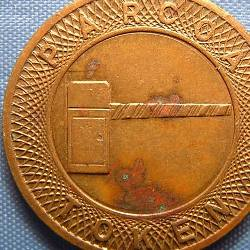usa aa gate G gate = long - parcoa = 18mm / token = 15mm USA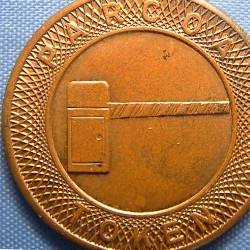usa aa gate I gate = long - parcoa = 17,3mm / token = 13mm USAusa aa gate A gate = short - parcoa = 13mm / token = 11mm USA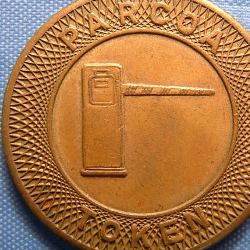usa aa gate B gate = short - parcoa = 14,3mm / token = 11,5mm / letters close to inner circl USA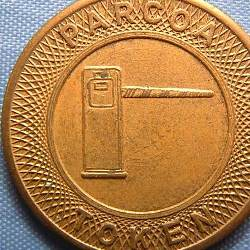usa aa gate C gate = short - parcoa = 13,6mm / token = 12,5mm / inner circle is thick USA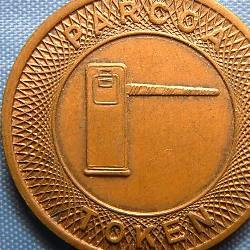usa aa gate D gate = short - parcoa = 14mm / token = 11,5mm / letters not close to inner circle USAusa aa gate E gate = long - parcoa = 15mm / token = 12,5mm USA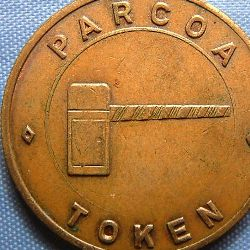usa aa gate F gate = long - parcoa = 17mm / token = 14mm == letter background is plain == 2 diamonds USA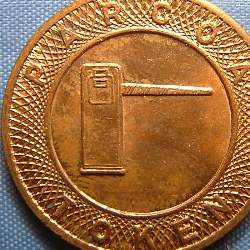usa aa gate H gate = short - parcoa = 20mm / token = 15,7mm USA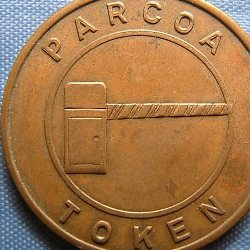usa aa gate J gate = long - parcoa = 16mm / token = 14,8mm == letter background is plain == no diamonds USA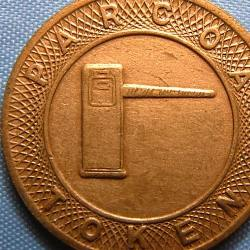usa aa gate L gate = short - parcoa = 20mm / token = 17,5mm USA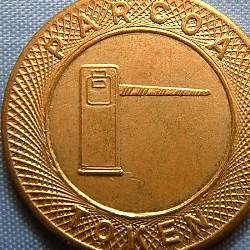usa aa gate M gate = short - parcoa = 16,5mm / token = 12,3mm USAusa aa gate N gate = short - parcoa = 15,8mm / token = 13,8mm USA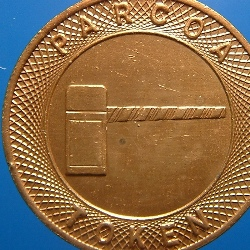usa aa gate Q gate = long - parcoa = 16,5mm / token = 13mm USAtest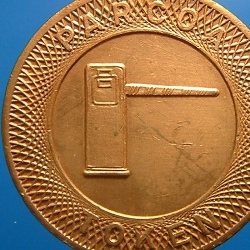usa aa gate K gate = short - parcoa = 14,9mm / token = 12,2mm USA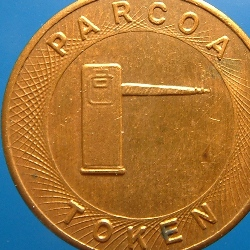usa aa gate S gate = short - parcoa = 16,4mm / token = 13,8mm USA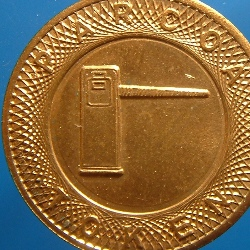usa aa gate R gate = short - parcoa = 19,5mm / token = 16,4mm USAlatv 3700 A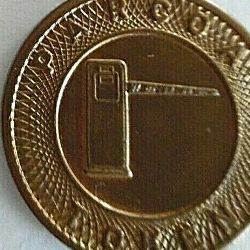usa aa gate O USA

1 2 3 .. -> .. 107

Pagenumber:

This website has last been updated: Saturday 2022-05-07 16:27:26 CET